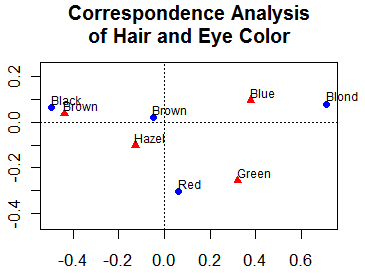This section describes more advanced statistical methods. This includes the discovery and exploration of complex multivariate relationships among variables. Links to appropriate graphical methods are also provided throughout. Basic statistics are described in the previous section.

It is difficult to order these topics in a straight-forward way. I have chosen the following (admittedly arbitrary) headings.

### Predictive models

Under predictive models, we have generalized linear models (include logistic regression, poisson regression, and survival analysis), discriminant function analysis (both linear and quadratic), and time series modeling.

### Latent Variable Models

This includes factor analysis (principal components, exploratory and confirmatory factor analysis), correspondence analysis, and multidimensional scaling(metric and nonmetric).

### Partitioning Methods

Cluster Analysis includes partitioning (k-means), hierarchical agglomerative, and model based approaches. Tree-Based methods (which could easily have gone under predictive models!) include classification and regression trees, random forests, and other partitioning methodologies.

### Other Tools

This section includes tools that are broadly useful including bootstrapping in R and matrix algebra programming (think MATRIX in SPSS or PROC IML in SAS).

## Going Further

Try the Supervised Learning in R course which includes an exercise with Random Forests.